Examples

Chapter 15 Class 9 Probability (Term 2)
Serial order wise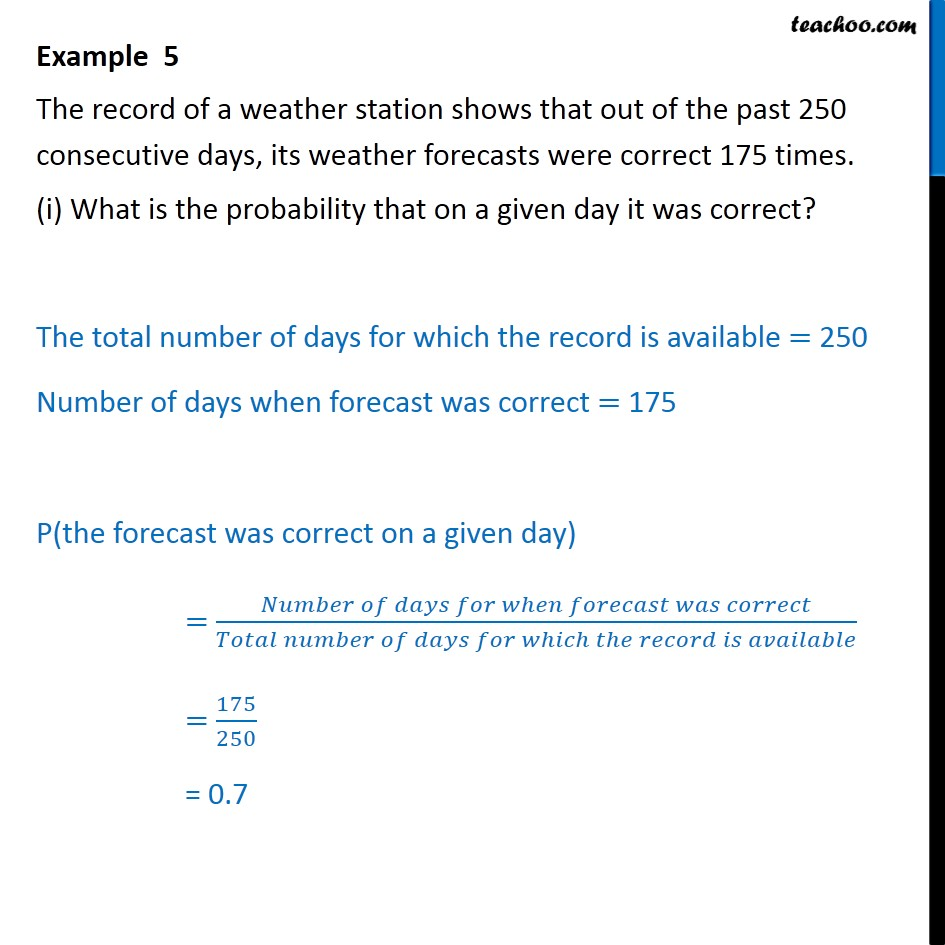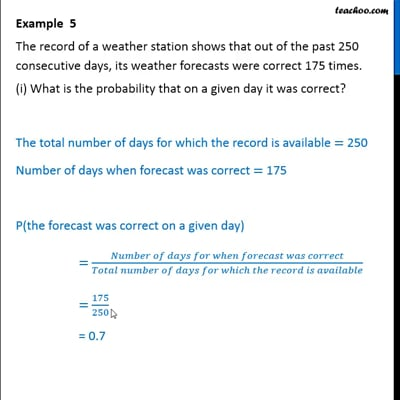This video is only available for Teachoo black users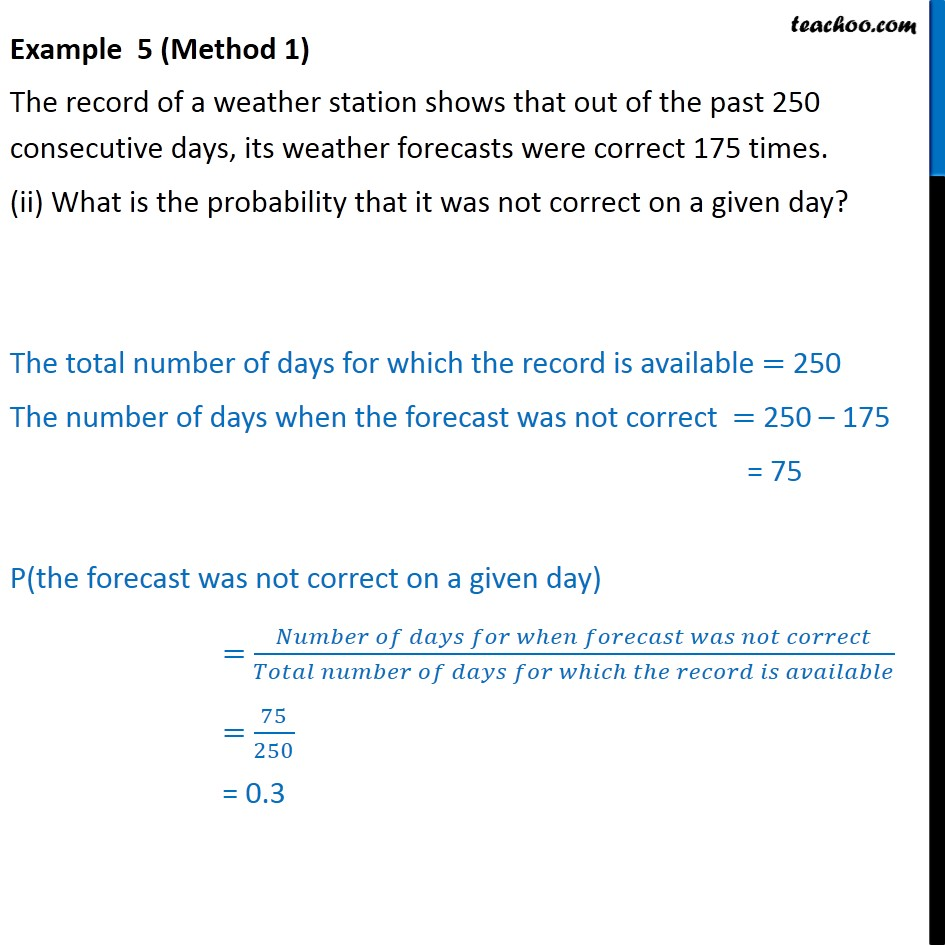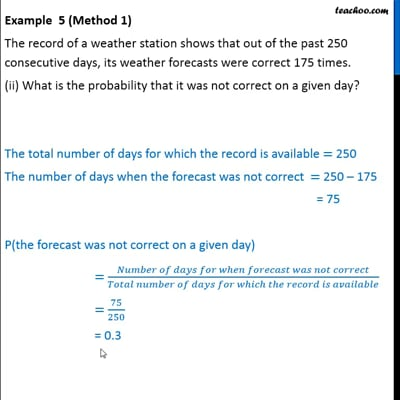This video is only available for Teachoo black users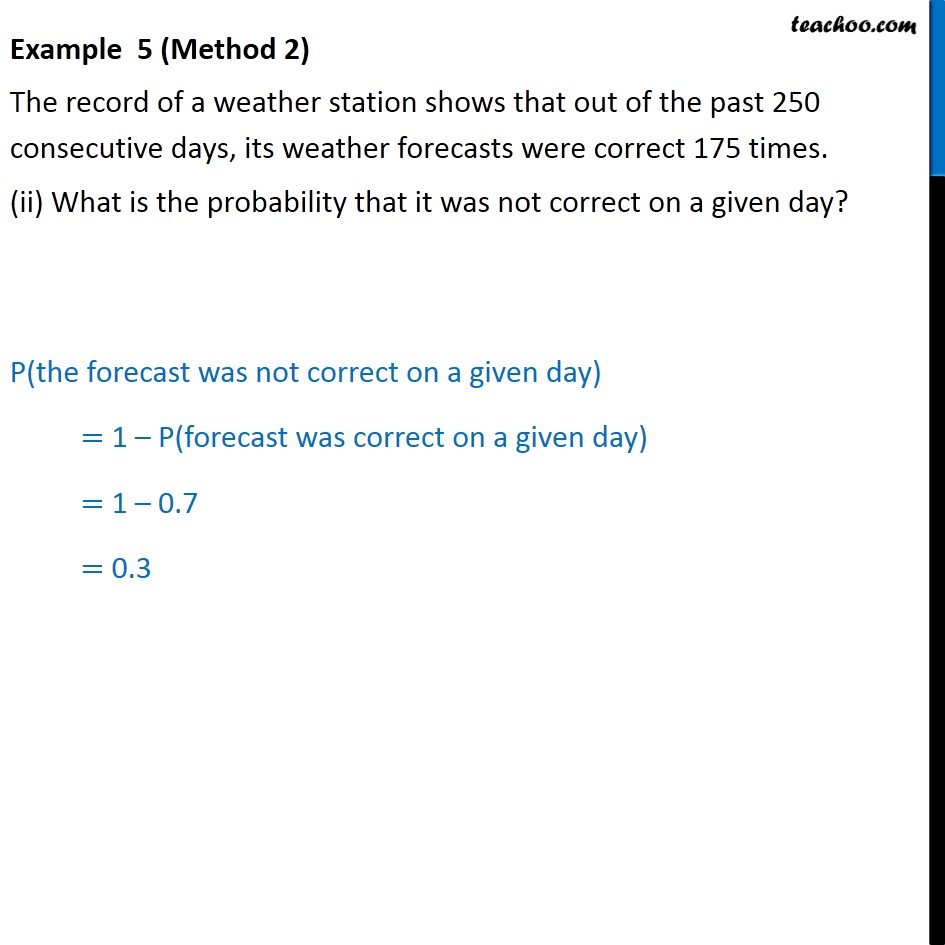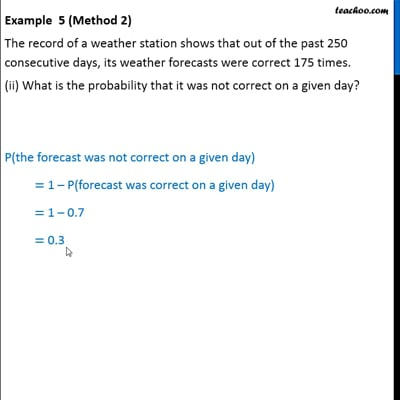This video is only available for Teachoo black users

### Transcript

Example 5 The record of a weather station shows that out of the past 250 consecutive days, its weather forecasts were correct 175 times. (i) What is the probability that on a given day it was correct? The total number of days for which the record is available = 250 Number of days when forecast was correct = 175 P(the forecast was correct on a given day) = (𝑁𝑢𝑚𝑏𝑒𝑟 𝑜𝑓 𝑑𝑎𝑦𝑠 𝑓𝑜𝑟 𝑤ℎ𝑒𝑛 𝑓𝑜𝑟𝑒𝑐𝑎𝑠𝑡 𝑤𝑎𝑠 𝑐𝑜𝑟𝑟𝑒𝑐𝑡)/(𝑇𝑜𝑡𝑎𝑙 𝑛𝑢𝑚𝑏𝑒𝑟 𝑜𝑓 𝑑𝑎𝑦𝑠 𝑓𝑜𝑟 𝑤ℎ𝑖𝑐ℎ 𝑡ℎ𝑒 𝑟𝑒𝑐𝑜𝑟𝑑 𝑖𝑠 𝑎𝑣𝑎𝑖𝑙𝑎𝑏𝑙𝑒) = 175/250 = 0.7 Example 5 (Method 1) The record of a weather station shows that out of the past 250 consecutive days, its weather forecasts were correct 175 times. (ii) What is the probability that it was not correct on a given day? The total number of days for which the record is available = 250 The number of days when the forecast was not correct = 250 – 175 = 75 P(the forecast was not correct on a given day) = (𝑁𝑢𝑚𝑏𝑒𝑟 𝑜𝑓 𝑑𝑎𝑦𝑠 𝑓𝑜𝑟 𝑤ℎ𝑒𝑛 𝑓𝑜𝑟𝑒𝑐𝑎𝑠𝑡 𝑤𝑎𝑠 𝑛𝑜𝑡 𝑐𝑜𝑟𝑟𝑒𝑐𝑡)/(𝑇𝑜𝑡𝑎𝑙 𝑛𝑢𝑚𝑏𝑒𝑟 𝑜𝑓 𝑑𝑎𝑦𝑠 𝑓𝑜𝑟 𝑤ℎ𝑖𝑐ℎ 𝑡ℎ𝑒 𝑟𝑒𝑐𝑜𝑟𝑑 𝑖𝑠 𝑎𝑣𝑎𝑖𝑙𝑎𝑏𝑙𝑒) = 75/250 = 0.3 Example 5 (Method 2) The record of a weather station shows that out of the past 250 consecutive days, its weather forecasts were correct 175 times. (ii) What is the probability that it was not correct on a given day? P(the forecast was not correct on a given day) = 1 – P(forecast was correct on a given day) = 1 – 0.7 = 0.3